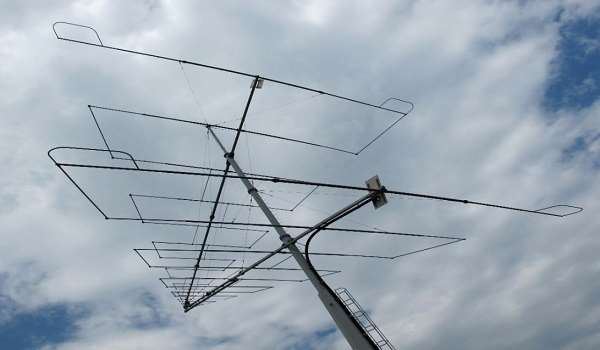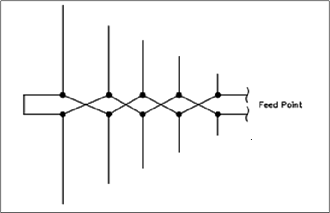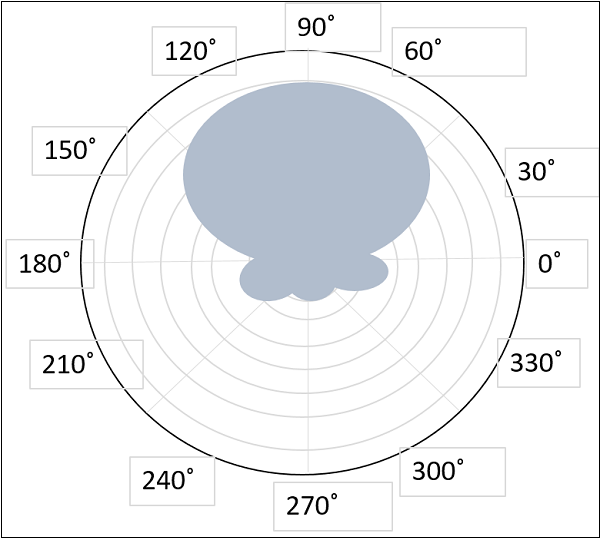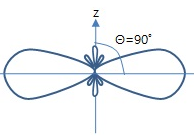# Antenna Theory - Log-periodic Antenna

The Yagi-Uda antenna is mostly used for domestic purpose. However, for commercial purpose and to tune over a range of frequencies, we need to have another antenna known as the Log-periodic antenna. A Log-periodic antenna is that whose impedance is a logarithamically periodic function of frequency.

### Frequency range

The frequency range, in which the log-periodic antennas operate is around 30 MHz to 3GHz which belong to the VHF and UHF bands.

## Construction & Working of Log-periodic Antenna

The construction and operation of a log-periodic antenna is similar to that of a Yagi-Uda antenna. The main advantage of this antenna is that it exhibits constant characteristics over a desired frequency range of operation. It has the same radiation resistance and therefore the same SWR. The gain and front-to-back ratio are also the same.The image shows a log-periodic antenna.

With the change in operation frequency, the active region shifts among the elements and hence all the elements will not be active only on a single frequency. This is its special characteristic.

There are several type of log-periodic antennas such as the planar, trapezoidal, zig-zag, V-type, slot and the dipole. The mostly used one is log-periodic dipole array, in short, LPDA.The diagram of log-periodic array is given above.

The physical structure and electrical characteristics, when observed, are repetitive in nature. The array consists of dipoles of different lengths and spacing, which are fed from a two-wire transmission line. This line is transposed between each adjacent pair of dipoles.

The dipole lengths and seperations are related by the formula −

$$\frac{R_{1}}{R_{2}} = \frac{R_{2}}{R_{3}} = \frac{R_{3}}{R_{4}} = T = \frac{l_{1}}{l_{2}} = \frac{l_{2}}{l_{3}} = \frac{l_{3}}{l_{4}}$$

Where

• т is the design ratio and т<1
• R is the distance between the feed and the dipole
• l is the length of the dipole.

The directive gains obtained are low to moderate. The radiational patterns may be Unidirectional or Bi-directional.

The Radiation pattern of log-periodic antenna can be of uni-directional or bi-directional, depending upon the log periodic structures.

For uni-directional Log-periodic antenna, the radiation towards shorter element is of considerable amount, whereas in forward direction, it is small or zero.The radiational pattern for uni-directional log-periodic antenna is given above.

For bi-directional Log-periodic antenna, the maximum radiation is in broad side, which is normal to the surface of the antenna.The figure given above shows the radiational pattern for a bi-directional log-periodic antenna.

The following are the advantages of Log-periodic antennas −

• The antenna design is compact.
• Gain and radiation pattern are varied according to the requirements.

The following are the disadvantages of Log-periodic antennas −

• External mount.
• Installation cost is high.

### Applications

The following are the applications of Log-periodic antennas −

• Used for HF communications.
• Used for particular sort of TV receptions.
• Used for all round monitoring in higher frequency bands.